# SSAT Upper Level Math : How to find the area of a parallelogram

## Example Questions

← Previous 1

### Example Question #53 : Geometry

A parallelogram has the base length ofand the altitude of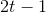. Give the area of the parallelogram.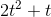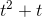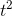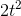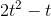Explanation:

The area of a parallelogram is given by: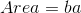Whereis the base length andis the corresponding altitude. So we can write: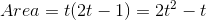### Example Question #54 : Geometry

A parallelogram has a base length ofwhich is 3 times longer than its corresponding altitude. The area of the parallelogram is 12 square inches. Give the.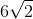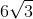Explanation:

Base length isso the corresponding altitude is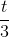.

The area of a parallelogram is given by:Where:is the length of any baseis the corresponding altitude

So we can write: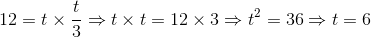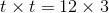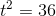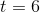### Example Question #55 : Geometry

The length of the shorter diagonal of a rhombus is 40% that of the longer diagonal. The area of the rhombus is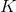. Give the length of the longer diagonal in terms of.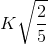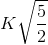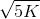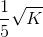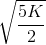Explanation:

Let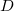be the length of the longer diagonal. Then the shorter diagonal has length 40% of this. Since 40% is equal to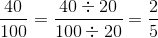, 40% ofis equal to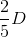.

The area of a rhombus is half the product of the lengths of its diagonals, so we can set up, and solve for, in the equation: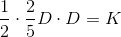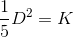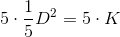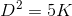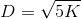### Example Question #56 : Geometry

The length of the shorter diagonal of a rhombus is two-thirds that of the longer diagonal. The area of the rhombus is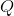square yards. Give the length of the longer diagonal, in inches, in terms of.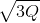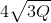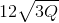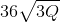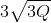Explanation:

Letbe the length of the longer diagonal in yards. Then the shorter diagonal has length two-thirds of this, or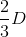.

The area of a rhombus is half the product of the lengths of its diagonals, so we can set up the following equation and solve for: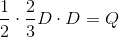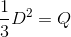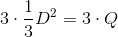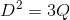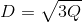To convert yards to inches, multiply by 36: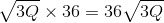### Example Question #57 : Geometry

The longer diagonal of a rhombus is 20% longer than the shorter diagonal; the rhombus has area. Give the length of the shorter diagonal in terms of.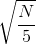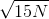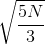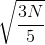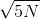Explanation:

Letbe the length of the shorter diagonal. If the longer diagonal is 20% longer, then it measures 120% of the length of the shorter diagonal; this is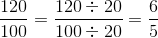of, or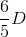.

The area of a rhombus is half the product of the lengths of its diagonals, so we can set up an equation and solve for: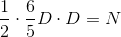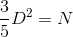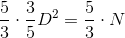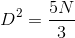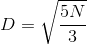### Example Question #1 : How To Find The Area Of A Parallelogram

Which of the following shapes is NOT a quadrilateral?

Rectangle

Square

Kite

Rhombus

Triangle

Triangle

Explanation:

A quadrilateral is any two-dimensional shape withsides. The only shape listed that does not havesides is a triangle.

### Example Question #2 : How To Find The Area Of A Parallelogram

What is the main difference between a square and a rectangle?

The number of sides they each have

Their angle measurments

Their side lengths

Their color

The sum of their angles

Their side lengths

Explanation:

The only difference between a rectangle and a square is their side lengths. A square has to haveequal side lengths, but the opposite side lengths of a rectangle only have to be equal.

### Example Question #3 : How To Find The Area Of A Parallelogram

What is the main difference between a triangle and a rectangle?

The volume

The length of the sides

The area

The color

The number of sides

The number of sides

Explanation:

Out of the choices given, the only characteristic used to describe shapes is the number of sides. A triangle hassides and a rectangle hassides.

### Example Question #4 : How To Find The Area Of A Parallelogram

Which two shapes have to haveright angles?

Square and Rectangle

Rectangle and Rhombus

Square and Rhombus

Square and Parallelogram

Rectangle and Parallelogram

Square and Rectangle

Explanation:

By definition, the only two quadrilaterals that have to haveright angles, are the square and the rectangle.

### Example Question #2 : Understand Categories And Subcategories Of Two Dimensional Figures: Ccss.Math.Content.5.G.B.3

Which of the shapes is NOT a quadrilateral?

Trapezoid

Rhombus

Rectangle

Hexagon

Square

A quadrilateral is asided shape. The only shape listed that does not havesides is a hexagon, which hassides.